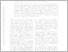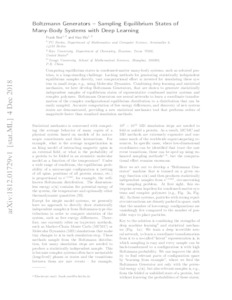Repository: Freie Universität Berlin, Math Department

# Boltzmann Generators: Sampling Equilibrium States of Many-Body Systems with Deep Learning

Noé, F. and Olsson, S. and Köhler, J. and Wu, H. (2019) Boltzmann Generators: Sampling Equilibrium States of Many-Body Systems with Deep Learning. Science, 365 (6457). eaaw1147.Preview

5MB

Official URL: https://dx.doi.org/10.1126/science.aaw1147

## Abstract

Computing equilibrium states in condensed-matter many-body systems, such as solvated proteins, is a long-standing challenge. Lacking methods for generating statistically independent equilibrium samples directly, vast computational effort is invested for simulating these system in small steps, e.g., using Molecular Dynamics. Combining deep learning and statistical mechanics, we here develop Boltzmann Generators, that are shown to generate statistically independent samples of equilibrium states of representative condensed matter systems and complex polymers. Boltzmann Generators use neural networks to learn a coordinate transformation of the complex configurational equilibrium distribution to a distribution that can be easily sampled. Accurate computation of free energy differences, and discovery of new system states are demonstrated, providing a new statistical mechanics tool that performs orders of magnitude faster than standard simulation methods.

Item Type: Article SFB1114 Preprint 12/2018 in arXiv:1812.01729 (https://arxiv.org/abs/1812.01729) Mathematical and Computer Sciences > Mathematics > Applied MathematicsMathematical and Computer Sciences > Artificial Intelligence > Machine Learning Department of Mathematics and Computer Science > Institute of Mathematics > Comp. Molecular Biology 2295 Silvia Hoemke 07 Feb 2019 12:54 21 Oct 2019 15:09

Repository Staff Only: item control page